5th of November, 2008

1. A point charge is in equilibrium if the force acting on it is 0, i.e. the electric field is zero at the position of the charge.

The equilibrium is stable if when the charge is displaced from the equilibrium position by a small distance, the forces acting on it push it back towards the equilibrium point (and not away from it).

Suppose that there is a stable equilibrium point in a static electric field. The criterion of stability requires that the electric field point inwards on every point of a surface enclosing the equilibrium point. This would mean that the surface integral of the electric field in non-zero on this surface—a result which contradicts that the equilibrium point is in vacuum, i.e. there are no charges inside the surface. Therefore, the original assumption, that there exists a stable equilibrium point, must be false.

2. We can use a method similar to that employed in the previous point. In this case, the force acting on a magnetic dipole is the negative gradient of its potential energy, i.e.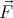= (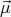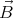), where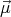is the dipole moment of the small magnet.
1. First, let us take the case when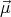is constant (its orientation is fixed), and calculate the divergence of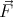. Choosing the z axis to be parallel with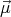,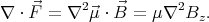But it can be proven that the Laplcaian of any component of the magnetic field is 0 in vaccum. Taking the curl of both sides of the equation ∇×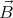= 0, and using that ∇⋅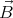= 0, we get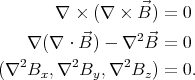The divergence of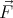is zero, therefore, in analogy with the case of the electric field, a small magnet (with fixed orientation) cannot have a stable equilibrium point in a magnetic field.

2. Now let us take the case when the dipole may rotate freely. If there is some friction (e.g. the magnet is moving in a viscuous medium), then, given sufficient time, the magnet will adopt an orientation that is parallel with the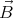field. Its potential energy will be Wd = -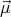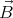= -μB. In a stable equilibrium point the potential energy has a minimum. To simplify the calculations, let us first consider the energy of a small piece of magnetizable material. Its magnetic moment is proportional to the magnetic field,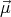= k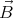, so its potential energy is Wm = -kB2. Since W m can be obtained from Wd by a monotonous tranformation, for a positive k, wherever Wm has a minimum, Wd has a minimum too. So the results obtained for a piece of magnetizable material (with k > 0) are applicable to a constant magnet too.

Let us calculate the divergence of the force acting on a piece of magnetizable material: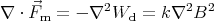But 2B2 can be shown to be positive or zero: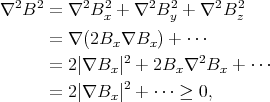and therefore ∇⋅m 0.

With a reasoning similar to the one used in the previous point it can be shown that a force field having ∇⋅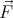0 only has unstable equilibrium points. (The integral over the Gaussian surface drawn around the equilibrium point is positive or zero, therefore there must exist at least some points on it where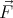is pointing outwards, or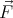must be zero everywhere.)

Therefore neihter a small piece of magnetic material with k > 0, nor a little magnet has stable equilibrium points in a static magnetic field. But for a diamagnet k < 0, i.e. the magnetic moment is always oriented antiparallel to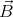, so a diamagnet does have stable equilibrium points.

1. Let us imagine that the atmosphere is divided into very thin horizontal layers, within which the index of refraction is constant.

Let ni denote the index of refraction of the ith layer from the ground, and φi denote the altitude of the star when viewed from that layer. Writing Snell’s law of refraction for the consecutive layers,

 n1 sin(π∕2 - φ1) = n2 sin(π∕2 - φ2) n2 sin(π∕2 - φ2) = n3 sin(π∕2 - φ3) … ni sin(π∕2 - φi) = ni+1 sin(π∕2 - φi+1) …
we see that n1 sin(π∕2 - φ1) = ni sin(π∕2 - φi) for any i. As we go very high up, the atmosphere gets thinner, so n approaches 1 and φ approaches φr. Thus the real altitude of the star can be obtained from the equation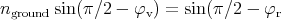2. Let the magnitude of the charge of the ions be q. The electrostatic energy of one ion the electric field of others is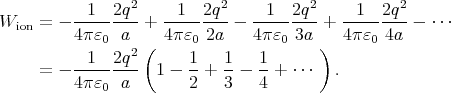The Taylor expansion of ln(1 + x) is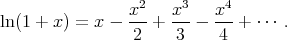Substituting x = 1 we find that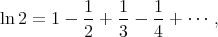and therefore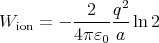Notice that if we add the energy of all ions in the crystal, we get double the energy of the complete crystal. (If we have only two ions, the energy of the complete system is the energy of one ion in the electric field of the other. Adding the energy of both gives twice this value.) So the electrostatic energy of the crystal per ion is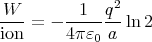3. Water is incompressible, so according to the continuity equation ∇⋅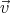= 0. Since ∇×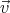= 0, there exists a scalar field ψ so that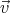= -∇ψ, and 2ψ = 0. This is completely analogous with electrostatics.

Now let us examine the problem from the reference frame moving together with the ball. The electrostatic analogy still holds in this reference frame. In this reference frame the ball is not moving, but the water is flowing past the ball. Since the water cannot flow into or out of a solid ball, on the surface of the ball it must be true that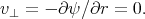(A)

(r is the radial coordinate measured from the centre of the ball.) Very far from the ball the flow is constant,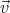=0 (where -v0 is the velocity of the ball in the original reference frame.)

How should the homogeneous flow field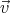0 be changed so that condition (A) will be satisfied? We need to find a field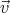which vanishes at infinity, cancels the normal component of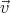0 on the surface of the sphere, and satisfies ∇⋅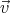= ∇×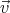= 0. The sum0 +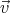will be the solution.

We have seen a similar problem in electrostatics: a conducting sphere placed in a homogeneous electric field. The difference is that there the tangential (and not normal) component of the electric field needed to be cancelled on the surface of the sphere. There we found that the distortion in the field caused by the sphere is a dipole field.

It turns out that a suitable chosen dipole field can cancel not only the tangential component of a homogeneous field on the surface of a sphere, but the normal component too. A dipole potential has the form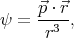where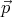is a constant vector, and the dipole field is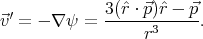Let us choose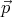so that it is parallel to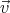0 and calculate the normal components of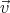0 and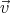at the surface of the sphere. We shall use spherical coordinates, with θ being the angle between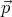and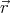. Let R be the radius of the sphere.

 v0⊥ =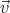0 ⋅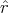= v0 cos θ v′⊥ =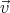′⋅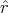=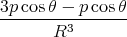=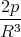cos θ
If p is chosen so that 2p∕R3 = -v 0 then the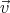will cancel the normal component of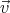0 on the surface of the sphere. The solution is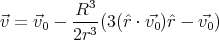in the reference frame moving together with the ball.

Back to the main page